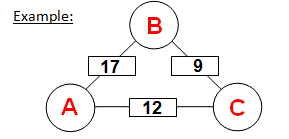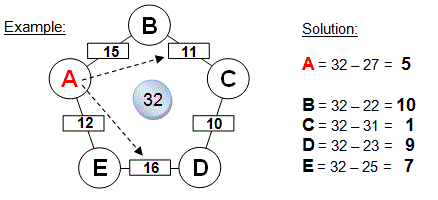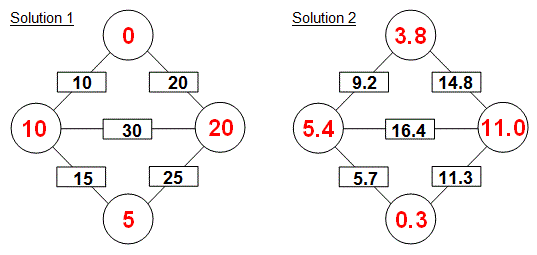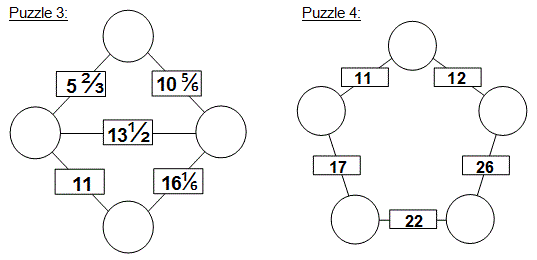Select PagePrintable version

In Addition Arithmagons the value of each square is the sum of the values in the circles or vertices on each side of it. Determine the value of each circle to complete the following puzzle.

Not sure where to start?  Look at the walkthrough examples first

### Walkthrough example one

An Arithmagon is defined as a polygon with numbers at its vertices which determine the numbers written on its edges. The following example is an triangular ‘addition’ type of Arithmagon puzzle.To solve this puzzle in algebraic terms, A + B = 17, B + C = 9 and C + A = 12.  Solving the 3 equations with 3 unknowns, the end result will be A = (17 + 9 + 12) /2 – 9 = 10 or = (17 – 9 + 12) /2 = 10, B = (17 + 9 + 12) /2 – 12 = 7 or = (17 + 9 – 12) /2 = 7 and C = (17 + 9 + 12) /2 – 17 or = (-17 + 9 + 12) /2 = 2.  In other words to solve this puzzle, simply add the three box values (ie., 17 + 9 + 12 = 38) and then divide by 2 (ie., 38 /2 = 19). That gives you a “center” number (19) for the triangle.  Then for each corner circle or vertice value, take the value in the opposite box to it and subtract it from the ‘center’ number and the solution again will be as follows:### Walkthrough example two

In the case of a pentagonal ‘addition’ Arithmagon diagram, the puzzle can be solve simply by adding the 5 box numbers and then divide by 2.  Again, that gives you a “center” number for the pentagonThen for each corner circle or vertice value, take the sum of the two boxes (not opposite or adjacent to it) and subtract it from the ‘center’ number.### Solution### Solution## Feedback

There are more than one way of doing these puzzles and may well be more than one answer.  Please let me and others know what alternatives you find by commenting below.  We also welcome general comments on the subject and any feedback you’d like to give.   If you have a question that need a response from me or you would like to contact me privately, please use the contact form.

## Get more puzzles!

If you’ve enjoyed doing the puzzles, consider ordering the book; 150+ of the best puzzles in a handy pocket sized format. Click here for full details.